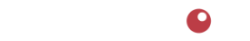# Calculators

Food Methods has developed several calculators

We've developed these calculators to help you use information to make better business decisions. These calculators are available to you to use free of charge. Food Methods does not save any information entered in the calculators.

## Margin Calculator

Calculates the % margin when cost and price are known

## Price Calculator (2-tier)

Calculates the price from cost and desired margin %. Uses a 2-tier model for Manufacturer, and Retailer.

## Price Calculator (3-tier)

Calculates the price from cost and desired margin %. Uses a 3-tier model for Manufacturer, Distributor, and Retailer.

## Breakeven Units Calculator

Calculates the number of units that must be sold during a period to avoid a loss.

## Price Increase Calculator

Calculates the price increase to account for periodic discounts.Portland, Oregon

503.234.7199

Notice | Privacy